Worksheets and No Prep Teaching Resources Math Worksheets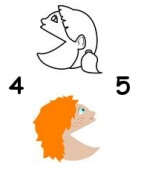Greater Than Less Than Handouts and Printables

>Greater> <Less< Than Puzzle

Comparing Numbers: Introduction to Greater Than
Comparing Numbers 0 to 9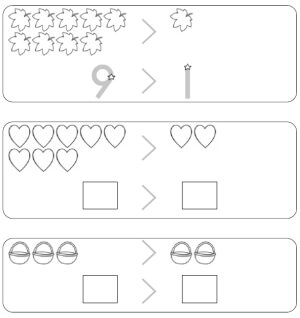Comparing Numbers 0 to 9 with Greater Than - All Trace (no blanks) Comparing Numbers 0 to 9 with Greater Than - All Trace (1 blank number) Comparing Numbers 0 to 9 with Greater Than - All Trace (1 blank number and 1 blank greater than) Comparing Numbers 0 to 9 with Greater Than - All Trace (all numbers blank) Comparing Numbers 0 to 9 with Greater Than - All Trace (all numbers blank and 1 blank greater than)

Comparing Numbers: Introduction to Less Than
Comparing Numbers 0 to 9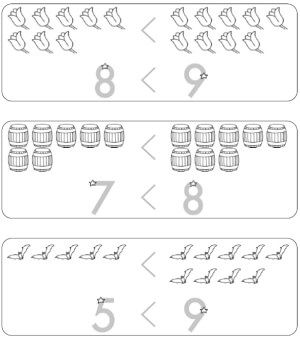Comparing Numbers 0 to 9 with Less Than - All Trace (no blanks) Comparing Numbers 0 to 9 with Less Than - All Trace (1 blank number) Comparing Numbers 0 to 9 with Less Than - All Trace (1 blank number and 1 blank less than) Comparing Numbers 0 to 9 with Less Than - All Trace (all numbers blank) Comparing Numbers 0 to 9 with Less Than - All Trace (all numbers blank and 1 blank less than)

Comparing Numbers: Mix of Greater or Less Than
Comparing Numbers 0 to 9

Coloring in Greater or Less Than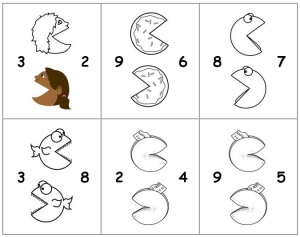Filling in Blanks - with Graphics and List of Numbers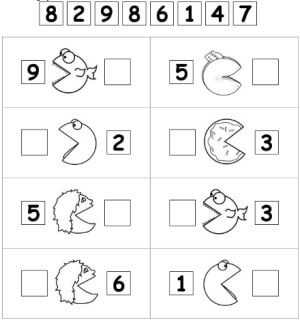Filling in Blanks - with Graphics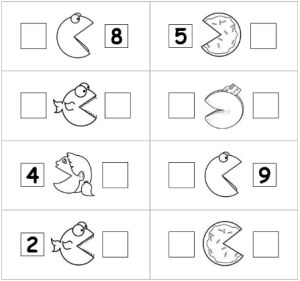Circle the Numbers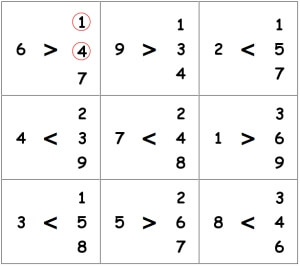Filling in Blanks - with some Graphics and some > and < signs - Fill-Ins to Use at Top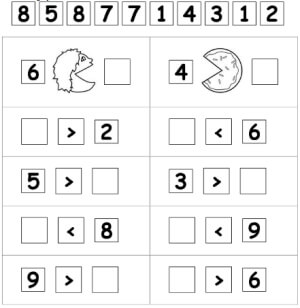Filling in Blanks - with some Graphics and some > and < signsGreater and Less Than: Make the Scale Balance
Easier: Maximum Sum of 19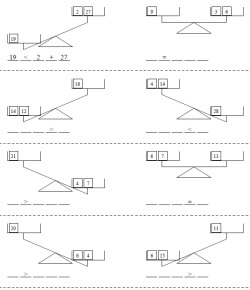Answer given for the first problem - the sign is given for all the problems Answer given for the first problem - the sign is given for the first four problems Answer given for the first problem No answers, but blank lines are shown for parts ___ ____ ____ ____ _____
Maximum Sum of 99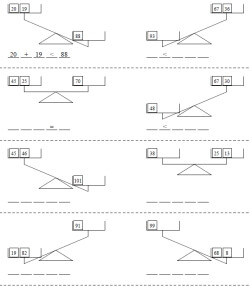Answer given for the first problem - the sign is given for all the problems Answer given for the first problem - the sign is given for the first four problems Answer given for the first problem No answers, but blank lines are shown for parts ___ ____ ____ ____ _____
Algebra Challenge - Maximum Sum of 19 - All balances are equal and there is one UNKNOWN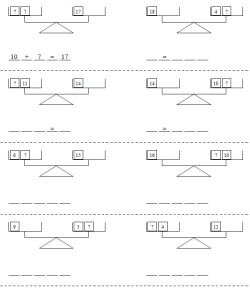Algebra Challenge: Answer given for the first problem - the sign is given for all the problems Algebra Challenge: Answer given for the first problem - the sign is given for the first four problems Algebra Challenge: Answer given for the first problem Algebra Challenge: No answers, but blank lines are shown for parts ___ ____ ____ ____ _____

Less Than/Greater Than Activity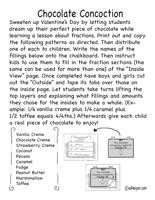Chocolate Concoctions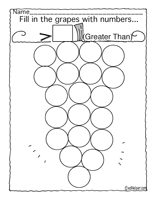Greater Than Grapes

Number and Letter Kissing Activity Lesson

More Activities and Printables
 Comparisons
 More
 More Than

Greater and Less Than Activity Book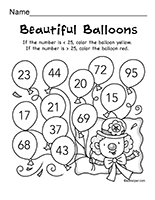Greater Less Than Activities

Bulletin Board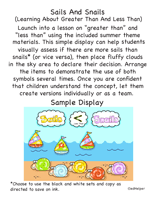Sails And Snails

Teacher Recommendations:  Worksheet and Lesson Plan Activity Ideas

Math

Money

Poetry

Word Search

Patterns

Weather

Comparing Numbers

Science

Time

Cause And Effect

Solar System

Book Reports

Shapes

More Activities, Lesson Plans, and Worksheets

Have a suggestion or would like to leave feedback?